Click to Chat

1800-1023-196

+91-120-4616500

CART 0

• 0

MY CART (5)

Use Coupon: CART20 and get 20% off on all online Study Material

ITEM
DETAILS
MRP
DISCOUNT
FINAL PRICE
Total Price: Rs.

There are no items in this cart.
Continue Shopping• Complete JEE Main/Advanced Course and Test Series
• OFFERED PRICE: Rs. 15,900
• View Details

```Revision Notes on Integers

Introduction to Integers

There are so many situations where we have to use negative numbers. Negative Numbers are the numbers with the negative sign. These numbers are less than zero.

Example

We use negative numbers to represent temperature.Where +10 shows 10° hotter than 0 and -10 shows 10° colder than 0.

Successor and Predecessor

If we move 1 to the right then it gives the successor of that number and if we move 1 to the left then it gives the predecessor of that number.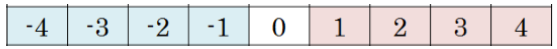Number
Predecessor
Successor

2
1
3

-8
-9
-7

-3
-4
-2

4
3
5

Tag me with a sign

In the case of accounting we use negative sign to represent the loss and positive to represent the profit.

In the case of sea level, we use a negative sign to represent the height of the place below the sea level and positive sign to represent the place above the sea level.

Integers

The collection of whole numbers and negative numbers together is called the Integers.

All the positive numbers are positive integers and all the negative numbers are negative integers. Zero is neither a positive nor a negative integer.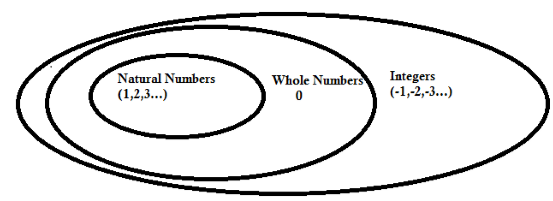Representation of Integers on Number Line

To represent the integers on a number line, first, we have to draw a line and mark a point zero on it.Then mark all the positive integers on the right side with the same distance as 1, 2, 3… and the entire negative numbers on the left side as -1,-2,-3…

Example

To mark (-7) we have to move 7 points to the left of zero.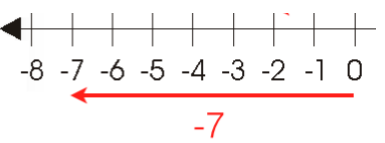Ordering of Integers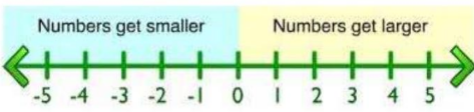From the above number line, we can see that as we go to the right side the numbers are getting larger and as we move to the left the numbers are getting smaller.

Hence, any number on the right side on the number line is greater than the number on its left.

Example

5 is to the right of 2 so 5>2.

4 is to the right of -2, so 4>-2.

-4 is to the left of -1, so -4 < -1.

Any positive integer is always greater than any negative integer.

Zero is less than every positive integer.

Zero is greater than every negative integer.

Zero doesn’t come in any of the negative and positive integers.

1. Addition of Two Positive Integers

If you have to add two positive integers then simply add them as natural numbers.

(+6) + (+7) = 6 + 7 = 13

2. Addition of Two Negative Integers

If we have to add two negative integers then simply add them as natural numbers and then put a negative sign on the answer.

(-6) + (-7) = - (6+7) = -13

3. Addition of One Negative and One Positive Integer

If we have to add one negative and one positive integer then simply subtract the numbers and put the sign of the bigger integer. We will decide the bigger integer ignoring the sign of the integers.

(-6) + (7) = 1 (bigger integer 7 is positive integer)

(6) + (-7) = -1(bigger integer 7 is negative integer)

Addition of Integers on a Number Line

1. Addition of Two Positive Integers

Example

Add 3 and 4 on the number line.

Solution

To add 3 and 4, first, we move 3 steps to the right of zero then again move 4 steps to the right from point 3.As we reached to the point 7, hence (+3) + (+4) = +7

This shows that the sum of two positive integers is always positive.

2. Addition of Two Negative Integers

Example

Add (-2) and (-5) using a number line.

Solution

To add (-2) and (-5), first we move 2 steps to the left of zero then again move 5 steps to the left of (-2).As we reached to the point (-7), hence (-2) + (-5) = -7.

This shows that the sum of two negative integers is always negative.

3. Addition of One Negative and One Positive Integer

a. If a positive integer is greater than the negative integer

To add (+6) and (-2), first we have to move 6 steps to the right from zero then move 2 steps to the left of point 6.As we reached to the point 4, hence (+6) + (-2) = +4

b. If a negative integer is greater than the positive integer

To add (-5) and (+4), first we have to move 5 steps to the left of zero then move 4 steps to the right from point (-5).As we reached to the point -1, hence (-5) + (+4) = (-1)

If we add numbers like (-7) and 7 then we get the result as zero. So these are called the Additive inverse of each other.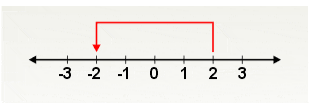If we add (-2) + (2), then first we move 2 steps to the left of zero then we move two steps to the right of (-2).so finally we reached to zero.

Hence, if we add the positive and negative of the same number then we get the zero.

Example

What is the additive inverse of 4 and (-8)?

Solution

The additive inverse of 4 is (-4).

The additive inverse of (-8) is 8.

Subtraction of Integers on Number Line

If we subtract an integer from another integer then we simply add the additive inverse of that integer.

(-3) – (-2) = (-3) + 2 = -1

(-3) – (+2) = (-3) + (-2) = -5

1. Subtraction of Two Positive Integers

Example

Subtract 2 from 5.

Solution

To subtract 2 from 5, first, we move 5 steps to the right from zero then move 2 steps back to the left.As we reached to 3 hence, 5 – (+2) = 5 – 2 = 3

2. Subtraction of Two Negative Integers

Example

Subtract (-12) from (-8).

Solution

To subtract (-12) from (-8), first, we have to move 8 steps to the left of zero then move 12 steps to the right of (-8).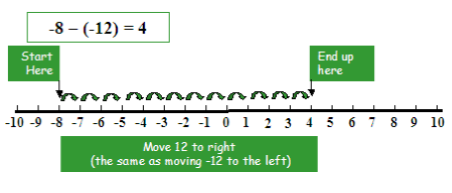As we reached to 4, hence (-8) – (-12) = (-8) + (12) = 4

3. Subtraction of One Negative and One Positive Integer

a. To subtract a positive integer from any other integer.

Example

Subtract 3 from (-4)

Solution

To subtract (-4) from (3), first, we have to move 4 steps to the left of zero then move 3 steps more to the left.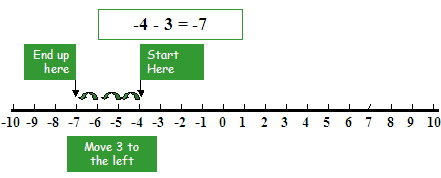As we reached to (-7), hence (-4) – (+3) = (-4) + (-3) = -7.

b. To subtract a negative integer from any other integer

Example

Subtract (-3) from (4)

Solution

To subtract (-3) from (4), first, we have to move 4 steps to the right of zero then move 3 steps more to the right.As we reached to (7), hence (4) – (-3) = (4) + (+3) = +7
```### Course Features

• 728 Video Lectures
• Revision Notes
• Previous Year Papers
• Mind Map
• Study Planner
• NCERT Solutions
• Discussion Forum
• Test paper with Video Solution